# TSA Bicoherence VI

LabVIEW 2014 Advanced Signal Processing Toolkit Help

Edition Date: June 2014

Part Number: 372656C-01

»View Product InfoDownload Help (Windows Only)

Owning Palette: Correlation and Spectral Analysis VIs

Computes the bicoherence of a univariate time series. The bicoherence is the normalized value of the bispectrum. Wire data to the Xt input to determine the polymorphic instance to use or manually select the instance.Note   You can use the TSA Bispectrum VI to compute the bispectrum of a univariate time series.

Example

Use the pull-down menu to select an instance of this VI.

 Select an instance TSA Bicoherence (Waveform)TSA Bicoherence (Array)

## TSA Bicoherence (Waveform)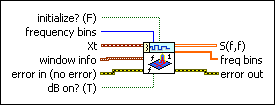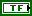initialize? specifies whether the input block of data is the first block. The default is FALSE, which means the input block of data is not the first block. When the input block of data is not the first block, this VI initializes the internal state of the block of data to the final state by using the previous call to this VI instance. When initialize? is TRUE, the input block of data is the first block and this VI initializes the internal state of the block of data to zero and processes one block of data.

To process consecutive blocks of data, set initialize? to TRUE for the first block and FALSE for all other blocks of data.frequency bins specifies the number of frequency bins for which this VI computes the bicoherence. The resulting bicoherence S(f,f) is a square 2D array with the size (frequency bins/2+1). The default is –1, which means this VI calculates an appropriate value for the frequency bins. Refer to the Details section of the TSA Bispectrum VI for information about how this VI calculates the frequency bins value.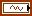Xt specifies the univariate time series.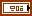window info specifies the information of the sliding window that divides the time series into subsequences.window specifies the time-domain window applied to the time series. Options include None (default), Hanning, Hamming, Blackman-Harris, Exact Blackman, Blackman, Flat Top, 4 Term B-Harris, 7 Term B-Harris, and Low Sidelobe.length specifies the length of the window. A large window generates a power spectral density (PSD) with small bias but results in a coarse PSD plot. A small window generates a smooth PSD plot but leads to large bias. The default is –1, which indicates that the window length equals the length of the input time series.overlap specifies the overlap, in percentage, of the moving window that this VI applies to the time series. This parameter determines how much data this VI reuses for the signal space matrix. A large overlap reduces the variance of the resulting power spectrum but increases computation time. The default is 50, which specifies that the overlap is half of the window length.error in describes error conditions that occur before this node runs. This input provides standard error in functionality.dB on? specifies whether this VI returns the S(f,f) in decibels or in a linear scale. If dB on? is TRUE, this VI returns the S(f,f) in decibels. If dB on? is FALSE, this VI returns the S(f,f) in a linear scale. The default is TRUE.S(f,f) returns the bicoherence S(f1, f2).freq bins returns the frequency bins at which this VI estimates the bicoherence.error out contains error information. This output provides standard error out functionality.

## TSA Bicoherence (Array)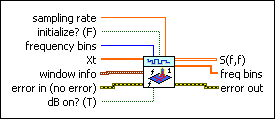sampling rate specifies the sampling rate, in hertz, of the univariate time series Xt. The default is 1.initialize? specifies whether the input block of data is the first block. The default is FALSE, which means the input block of data is not the first block. When the input block of data is not the first block, this VI initializes the internal state of the block of data to the final state by using the previous call to this VI instance. When initialize? is TRUE, the input block of data is the first block and this VI initializes the internal state of the block of data to zero and processes one block of data.

To process consecutive blocks of data, set initialize? to TRUE for the first block and FALSE for all other blocks of data.frequency bins specifies the number of frequency bins for which this VI computes the bicoherence. The resulting bicoherence S(f,f) is a square 2D array with the size (frequency bins/2+1). The default is –1, which means this VI calculates an appropriate value for the frequency bins. Refer to the Details section of the TSA Bispectrum VI for information about how this VI calculates the frequency bins value.Xt specifies the univariate time series.window info specifies the information of the sliding window that divides the time series into subsequences.window specifies the time-domain window applied to the time series. Options include None (default), Hanning, Hamming, Blackman-Harris, Exact Blackman, Blackman, Flat Top, 4 Term B-Harris, 7 Term B-Harris, and Low Sidelobe.length specifies the length of the window. A large window generates a power spectral density (PSD) with small bias but results in a coarse PSD plot. A small window generates a smooth PSD plot but leads to large bias. The default is –1, which indicates that the window length equals the length of the input time series.overlap specifies the overlap, in percentage, of the moving window that this VI applies to the time series. This parameter determines how much data this VI reuses for the signal space matrix. A large overlap reduces the variance of the resulting power spectrum but increases computation time. The default is 50, which specifies that the overlap is half of the window length.error in describes error conditions that occur before this node runs. This input provides standard error in functionality.dB on? specifies whether this VI returns the S(f,f) in decibels or in a linear scale. If dB on? is TRUE, this VI returns the S(f,f) in decibels. If dB on? is FALSE, this VI returns the S(f,f) in a linear scale. The default is TRUE.S(f,f) returns the bicoherence S(f1, f2).freq bins returns the frequency bins at which this VI estimates the bicoherence.error out contains error information. This output provides standard error out functionality.

## Example

Refer to the Bicoherence Analysis VI in the labview\examples\Time Series Analysis\TSAGettingStarted directory for an example of using the TSA Bicoherence VI.